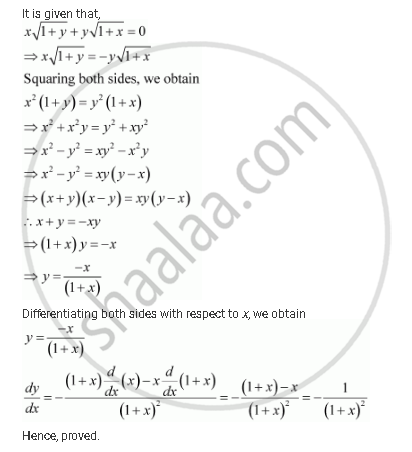Share

# If Xsqrt(1+Y) + Ysqrt(1+X) = 0, For, −1 < X <1, Prove that Dy/Dx = 1/(1+ X)^2 - CBSE (Commerce) Class 12 - Mathematics

ConceptDerivatives of Inverse Trigonometric Functions

#### Question

if xsqrt(1+y) + ysqrt(1+x) = 0, for, −1 < x <1, prove that dy/dx = 1/(1+ x)^2

#### SolutionIs there an error in this question or solution?

#### APPEARS IN

NCERT Solution for Mathematics Textbook for Class 12 (2018 to Current)
Chapter 5: Continuity and Differentiability
Q: 14 | Page no. 191

#### Video TutorialsVIEW ALL 

Solution If Xsqrt(1+Y) + Ysqrt(1+X) = 0, For, −1 < X <1, Prove that Dy/Dx = 1/(1+ X)^2 Concept: Derivatives of Inverse Trigonometric Functions.
S